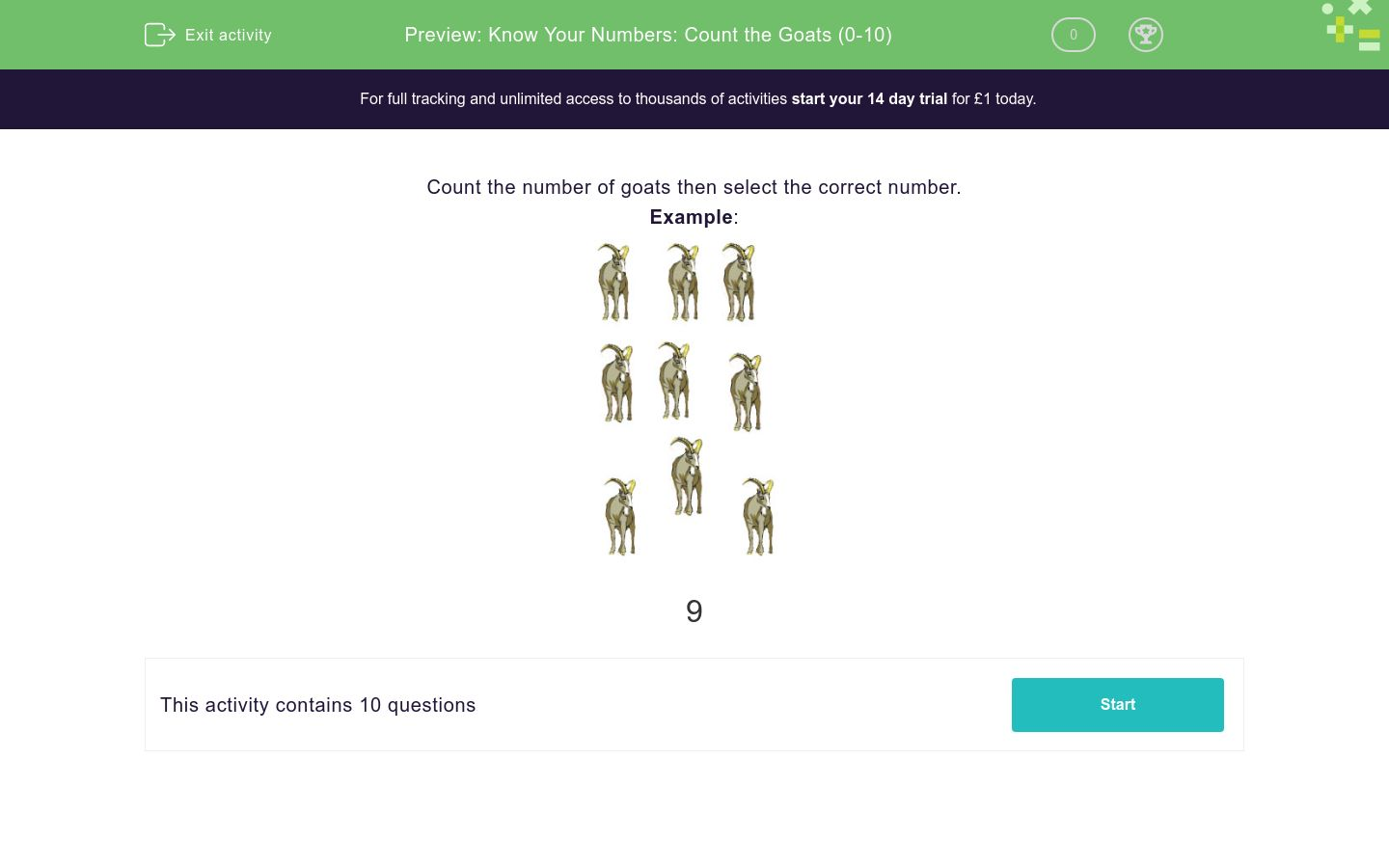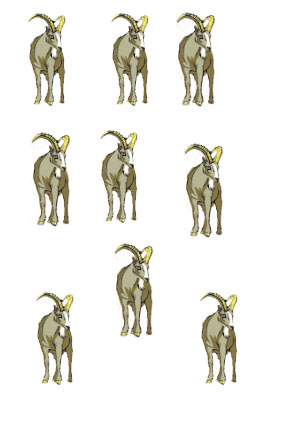# Know Your Numbers: Count the Goats (0-10)

In this worksheet, students count accurately within the 0-10 number range and then select the correct number.Key stage:  KS 1

Curriculum topic:   Number: Number and Place Value

Curriculum subtopic:   Count to 100

Difficulty level:### QUESTION 1 of 10

Count the number of goats then select the correct number.

Example:9

Count the number of goats then select the correct number.10

9

8

7

6

5

4

3

2

1

Count the number of goats then select the correct number.1

2

3

4

5

6

7

8

9

10

Count the number of goats then select the correct number.10

9

8

7

6

5

4

3

2

1

Count the number of goats then select the correct number.10

9

8

7

6

5

4

3

2

1

Count the number of goats then select the correct number.1

2

3

4

5

6

7

8

9

10

Count the number of goats then select the correct number.10

9

8

7

6

5

4

3

2

1

Count the number of goats then select the correct number.1

2

3

4

5

6

7

8

9

10

Count the number of goats then select the correct number.10

9

8

7

6

5

4

3

2

1

Count the number of goats then select the correct number.1

2

3

4

5

6

7

8

9

10

Count the number of goats then select the correct number.10

9

8

7

6

5

4

3

2

1

• Question 1

Count the number of goats then select the correct number.7
EDDIE SAYS
There are 7 goats.
• Question 2

Count the number of goats then select the correct number.9
EDDIE SAYS
There are 9 goats.
• Question 3

Count the number of goats then select the correct number.5
EDDIE SAYS
There are 5 goats.
• Question 4

Count the number of goats then select the correct number.8
EDDIE SAYS
There are 8 goats.
• Question 5

Count the number of goats then select the correct number.4
EDDIE SAYS
There are 4 goats.
• Question 6

Count the number of goats then select the correct number.2
EDDIE SAYS
There are 2 goats.
• Question 7

Count the number of goats then select the correct number.3
EDDIE SAYS
There are 3 goats.
• Question 8

Count the number of goats then select the correct number.1
EDDIE SAYS
There is 1 goat.
• Question 9

Count the number of goats then select the correct number.10
EDDIE SAYS
There are 10 goats.
• Question 10

Count the number of goats then select the correct number.7
EDDIE SAYS
There are 7 goats.
---- OR ----

Sign up for a £1 trial so you can track and measure your child's progress on this activity.

### What is EdPlace?

We're your National Curriculum aligned online education content provider helping each child succeed in English, maths and science from year 1 to GCSE. With an EdPlace account you’ll be able to track and measure progress, helping each child achieve their best. We build confidence and attainment by personalising each child’s learning at a level that suits them.

Get started